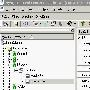# 用Perl的WriteExcel从数据库生成电子数据表格文件

use DBI;

# connect to the local database

my \$dbh = DBI-connect('dbi:Oracle:','scott','tiger') || die \$DBI::errstr;

print "Generating Excel Spreadsheet\n";

my \$workbook = new Spreadsheet::WriteExcel "emp.xls";

my \$worksheet = \$workbook-add_worksheet();

my \$fmt = {

bottom=2,bg_color='silver'),

num_format='\$#,##0.00',align='right'),

num_format='\$#,##0.00',align='right',bold=1,

top=2),

};

print "Querying Employees\n";

my \$sth = \$dbh-prepare('select * from emp') || die \$DBI::errstr;

my \$rc = \$sth-execute;

my \$r = 1;

\$r++;

while (my \$row = \$sth-fetchrow_hashref)

{

\$worksheet-write(\$r-1,0,\$row-{ENAME},\$fmt-{ENAME});

\$worksheet-write(\$r-1,1,\$row-{SAL},\$fmt-{MONEY});

\$worksheet-write(\$r-1,2,\$row-{COMM},\$fmt-{MONEY});

\$worksheet-write(\$r-1,3,"=B\$r+C\$r",\$fmt-{MONEY});

\$r++;

}

\$worksheet-write(\$r-1,0,'',\$fmt-{TOTAL});

\$worksheet-write(\$r-1,1,'=SUM(B2:B'.(\$r-1).')',\$fmt-{TOTAL});

\$worksheet-write(\$r-1,2,'=SUM(C2:C'.(\$r-1).')',\$fmt-{TOTAL});

\$worksheet-write(\$r-1,3,"=B\$r+C\$r",\$fmt-{TOTAL});

print "Processed " . (\$r-2) ." rows\n";

\$sth-finish;

· 湖北宜昌三峡坝区水面惊现神秘动物

· 什么是语段？语段的类型以及和句群、段落的区别与联系是什么？

· 十八部好看的赌石类小说

许多商业最终用户更喜欢使用目前流行的电子数据表格程序（如Microsoft Excel），而不是数据库功能或应用程序。尽管Excel可以用ODBC及相关技术来提取储存在Oracle数据库当中的数据，但想让用户建立一个环境来直接...查看完整版>>用Perl的WriteExcel从数据库生成电子数据表格文件

Hibernate技巧（1）：利用配置文件编写程序生成数据库
目前很多人使用Hibernate作为持久层，假如我们已经写了配置文件poweracl.hbm.XML，则不必再费劲写SQL的DDL。除了利用工具SchemaEXPort之外，还可以编写程序来自动初始化数据库，并且生成SQL DDL。(1)Hibernate配...查看完整版>>Hibernate技巧（1）：利用配置文件编写程序生成数据库非凡说明：希望朋友们在引用尊重版权，因为这是对像我这样的朋友的最大尊重！况且这是我2006的处女贴,也希望对大家有点用，工资狂飙升！！1. 首先是预备开源包，到http://jdom.org.下载，当前最新版本是jdom-...查看完整版>>利用jdom实现读取数据库生成XML及读取XML文件插入数据库

Hibernate:利用配置文件生成数据库